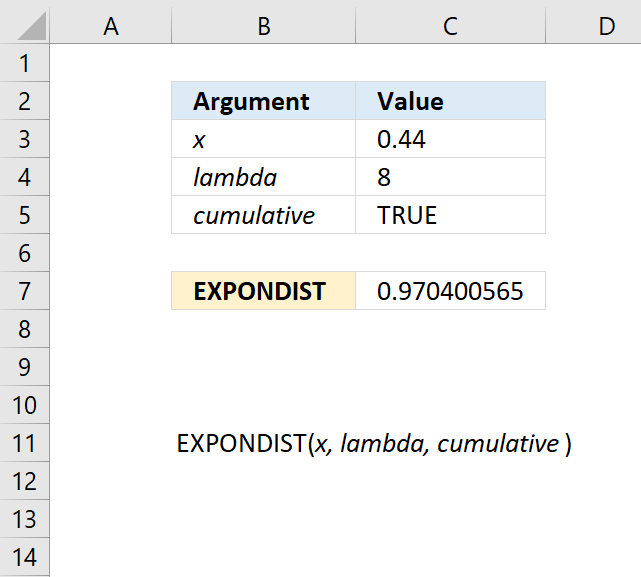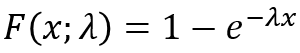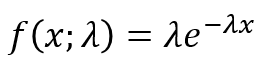Author: Oscar Cronquist Article last updated on October 09, 2018The EXPONDIST function calculates the exponential distribution representing an outcome in the form of probability.

This function is in the compatibility category and has been replaced by the EXPON.DIST function which was introduced in Excel 2010.

Formula in cell C7:

=EXPONDIST(C3,C4,C5)

### Excel Function Syntax

EXPONDIST(x,lambda,cumulative)

### Arguments

 x Required. lambda Required. cumulative Required. A boolean value. TRUE - cumulative distribution function FALSE - probability density function

The EXPONDIST function returns

• #VALUE! error value if lambda or x is non-numeric.
• #NUM! error value if:
• x < 0 (zero)
• lambda <= 0 (zero)

The general equation to calculate the cumulative distribution function:The general equation to calculate the probability density distribution: